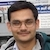# NAEST 2018 Screening Test: Convection CurrentBy

A cardboard box is fitted with two chimneys. A convection current is set-up when a lighted candle is placed below one of the chimneys. You can see the movement of air by using smoke. This experiment explains the reason behind the convection current. The pressure and air density at four points A, B, C and D (see figure) are analysed.

Question: The pressures of air at points A, B, C, D are $p_A$, $p_B$, $p_C$ and $p_D$. The air densities at these points are $\rho_A$, $\rho_B$, $\rho_C$ and $\rho_D$. Select the correct options(s)

1. $p_A > p_D$
2. $p_B > p_C$
3. $p_A > p_B$
4. $\rho_B > \rho_A$

Solution: The air in the vicinity of point A (above the candle) gets heated up due to the lighted candle. The rise in air temperature in this region increases the average speed of the air molecules and intramolecular separation between them. This leads to increase in the air pressure and a decrease in air density.The points C and D are exposed to the atmosphere. The pressure at these points will be approximately equal to the atmospheric pressure.

The air starts rising up from the point A to the point D due to the pressure difference between these points. To fill the gap at A, the air from the surrounding point B rushes to point A. This movement of air from B reduces the pressure at B. The air starts moving from C to B due to this pressure difference. This process sets up a convection current in which air moves from A to D to C to B in a cycle.

In summary, $p_A>p_D$ and $\rho_B>\rho_A$.